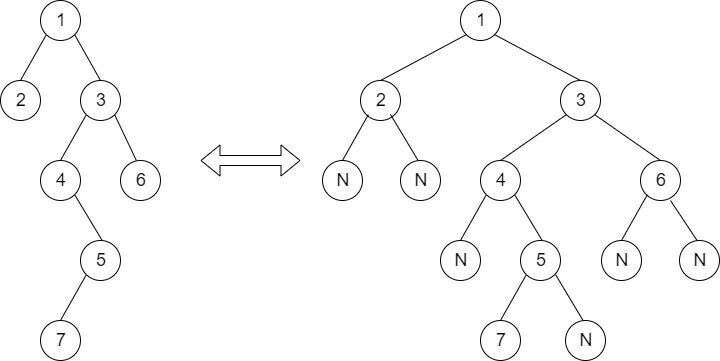Timer is Running

Extreme nodes in alternate order
##### Submissions: 7686   Accuracy: 46.34%   Difficulty: Medium   Marks: 4
Associated Course(s):   Amazon SDE Test Series

Given a binary tree, print nodes of extreme corners of each level but in alternate order.

Example:For above tree, the output is

1 2 7 8 31
– print rightmost node of 1st level
– print leftmost node of 2nd level
– print rightmost node of 3rd level
– print leftmost node of 4th level
– print rightmost node of 5th level

Input Format:
First line of input contains the number of test cases T. For each test case, there will be only a single line of input which is a string representing the tree as described below:

1. The values in the string are in the order of level order traversal of the tree where, numbers denotes node values, and a character “N” denotes NULL child.

2. For example:For the above tree, the string will be: 1 2 3 N N 4 6 N 5 N N 7 N

Output:
The function should print extreme nodes in alternte manner.

You don't have to take any input. Just complete the function printExtremeNodes() that takes root node as paramter  and print the answer. New line will be added by the driver code only.
Expected Time Complexity: O(N).
Expected Auxiliary Space: O(N).

Constraints:
1 <=T<= 30
1 <=Number of nodes<= 100
1 <=Data of a node<= 1000

Example:
Input:

1
1 2 3

Output:
1 2

Explanation:
First case represents a tree with 3 nodes and 2 edges where root is 1, left child of 1 is 3 and right child of 1 is 2.

Note:The Input/Ouput format and Example given are used for system's internal purpose, and should be used by a user for Expected Output only. As it is a function problem, hence a user should not read any input from stdin/console. The task is to complete the function specified, and not to write the full code.

#### ** For More Input/Output Examples Use 'Expected Output' option **

Contributor: Harshit Sidhwa
Author: kartik

If you have purchased any course from GeeksforGeeks then please ask your doubt on course discussion forum. You will get quick replies from GFG Moderators there.